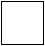Skill 4C
Fact Families

What is a fact family you ask?  Well, a fact family is simply how 3 numbers can be used together in a different order to make two separate addition and two separate subtraction problems (four altogether).  Sound confusing? Of course it is, but that's why we're here!  All you are doing is changing the order of the numbers in the problem to accomplish this.  For example, if we want to make a fact family using the numbers 2,3 and 5, we would make these four different problems:
2+3=5, 3+2=5, 5-3=2 and 5-2=3.

See how simple that was? As the term Fact Family is used frequently in 2nd Grade math textbooks we need to make sure this concept is understood.

Complete the fact family for the numbers

7, 3, and 10+=-=+=-=